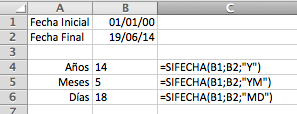# Calculate Working Time in Excel

Learn how to calculate the working age of any employee with this simple formula for calculating years, months and days worked.To calculate the age of any employee (or your own) you will need the initial and final dates (or the date today if they are still working with you, which you can easily find out using the code TODAY). By subtracting the dates you will find out the number of total days worked. It’s simple, right? You can learn how to calculate the difference between two dates here.

However, the complication comes when instead of days you want to know the amount of time in years, months or only working days. To do this calculation you have to take into account factors such as leap years and the duration of months (you can’t simply divide by 365).

## Calculating time worked in years, months and days

To be able to calculate a working age in terms of years, months and days, you will need to use the Excel code DATEDIF.

This function is very simple to use. All you have to do is input the initial date, the final date and in what measure you would like your results:

`DATEDIF(initial date, final date, years/months)`

If you would like to know the amount of time in the format of days, mark the unit “d” within the equation:

`DATEDIF(01/01/2000,19/07/2014,"d") = 5283`However, if you want to obtain results that are divided into years, months and days then you will need to carry out 3 different calculations, each with their own criteria:

• “Y” => to get the number of complete years between the two dates
• “YM” => to get the number of complete months between the two dates, deducting the complete years
• “MD” => to get the number of days between the two dates, deducting the complete years and months